Kernel Density Estimate with product approximation using multiscale Gibbs sampling

# JuliaRobotics/KernelDensityEstimate.jl

Switch branches/tags
Nothing to show

A tag already exists with the provided branch name. Many Git commands accept both tag and branch names, so creating this branch may cause unexpected behavior. Are you sure you want to create this branch?

## Files

Failed to load latest commit information.
Type
Name
Commit time

# KernelDensityEstimate.jl

Kernel Density Estimation with product approximation using multiscale Gibbs sampling.

All code is implemented in native Julia, including plotting. The main focus of this module is the ability to take the product between multiple KDEs, and makes this module unique from other KDE implementations. This package also supports n-dimensional KDEs. Please see examples below for details. The implementation is already fairly optimized from a symbolic standpoint and is based on work by:

``````Sudderth, Erik B.; Ihler, Alexander, et al. "Nonparametric belief propagation." Communications of the ACM 53.10 (2010): 95-103.
``````

## Installation

In Julia 1.0 and above:

`] add KernelDensityEstimate`

## Plotting Functions

The plotting functions for this library have been separated into KernelDensityEstimatePlotting.jl. Plotting functionality uses Gadfly. Comments welcome.

# Examples

Bring the module into the workspace

```using KernelDensityEstimate
# Basic one dimensional examples
# using leave-one-out likelihood cross validation for bandwidth estimation
p100 = kde!([randn(50);10.0.+2*randn(50)])
p2 = kde!([0.0;10.0],[1.0]) # multibandwidth still to be added
p75 = resample(p2,75)

# bring in the plotting functions
using KernelDensityEstimatePlotting
plot([p100;p2;p75],c=["red";"green";"blue"]) # using Gadfly under the hood```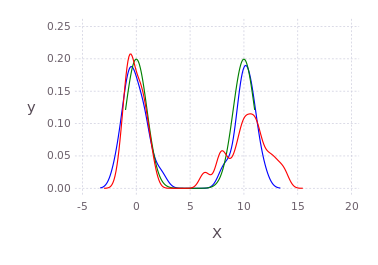Multidimensional example

```pd2 = kde!(randn(3,100));
@time pd2 = kde!(randn(3,100)); # defaults to loocv
pm12 = marginal(pd2,[1;2]);
pm2 = marginal(pm12,);
plot(pm2);```

Multiscale Gibbs product approximation example

```p = kde!(randn(2,100))
q = kde!(2.0.+randn(2,100))
dummy = kde!(rand(2,100),[1.0]);
mcmciters = 5
pGM, = prodAppxMSGibbsS(dummy, [p;q], nothing, nothing, Niter=mcmciters)
pq = kde!(pGM)
pq1 = marginal(pq,)
Pl1 = plot([marginal(p,);marginal(q,);marginal(pq,)],c=["red";"green";"black"])```

Direct histogram of points from the product

```using Gadfly
draw(PDF("product.pdf",15cm,8cm),hstack(Pl1,Pl2))```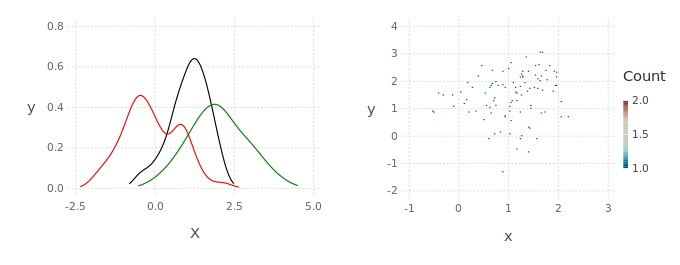KDE product between non-gaussian distributions

```using Distributions
p = kde!(rand(Beta(1.0,0.45),300));
q = kde!(rand(Rayleigh(0.5),100).-0.5);
dummy = kde!(rand(1,100),[1.0]);
pGM, = prodAppxMSGibbsS(dummy, [p;q], nothing, nothing, Niter=5)
pq = kde!(pGM)
plot([p;q;pq],c=["red";"green";"black"])```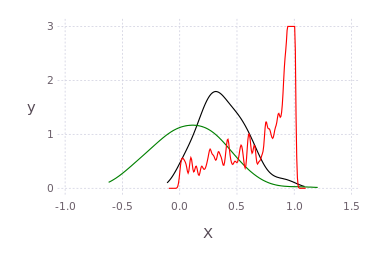Draw multidimensional distributions as marginalized 2D contour plots

```axis=[[-5.0;5]';[-2.0;2.0]';[-10.0;10]';[-5.0;5]']
draw(PDF("test.pdf",30cm,20cm),
plot( kde!(randn(4,200)) ) )

N=200;
pts = [2*randn(1,N).+3;
[2*randn(1,round(Int,N/2))'.+3.0;2*randn(1,round(Int,N/2))'.-3.0]';
2*randn(2,N).+3];
p, q = kde!(randn(4,100)), kde!(pts);
draw(PNG("MultidimPlot.png",15cm,10cm),
plot( [p*q;p;q],c=["red";"black";"blue"], axis=axis, dims=2:4,dimLbls=["w";"x";"y";"z"], levels=4) )```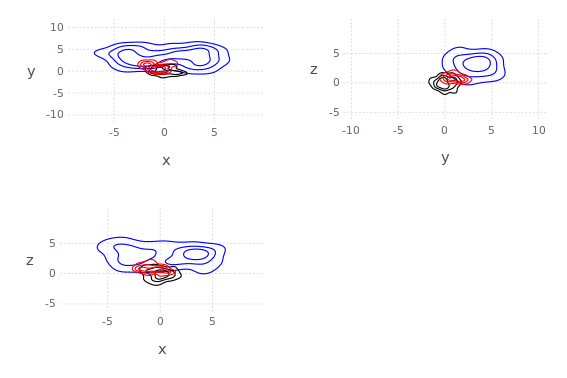```# or draw product natively
draw(PNG("MultidimPlotProd.png",10cm,7cm),
plot( p*q, axis=axis, dims=[2;4],dimLbls=["w";"x";"y";"z"]) )```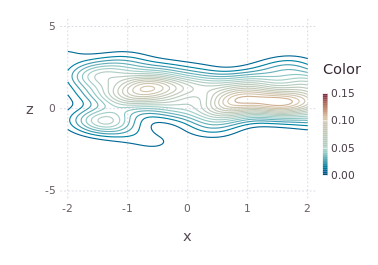# Contributors

The original C++ kde package was written by Alex Ihler and Mike Mandel in 2003, and has be rewritten in Julia and continuously modified by Dehann Fourie since.

Thank you to contributors and users alike, comments and improvements welcome according to JuliaLang and JuliaRobotics standards.

Kernel Density Estimate with product approximation using multiscale Gibbs sampling

v0.5.11 Latest
Aug 19, 2022

## Packages 0

No packages published